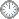# 在方法上下功夫绝不会白费。

This is an in-mail from TYUST.
新入の者--> What is going on ? (redirected)new

(接前：05 04 02) 转到命题 5.9 的证明.
Step2. Let ψ: W --> X be a log resolution of (X, B + tL) so that T is a divisor on W.
---- 以(X, B + tL) 为参照做回拉.
---- 这是要“造相”的征兆/节奏.
.
Define a boundary Γw = (1 + v)(B~ + tL~) + (1 - eps'/4)ΣEi + (1 - eps')T where Ei are the exceptional divisors of ψ other than T, and ~ denotes birational transform.
---- 定义边界Γw, 但这仅是表象.
---- 实际上Γw是构造出来的(参Pro.5.7,Step4).
(待温习时再仔细推敲).
---- 此处有个变化 (1 - a)T -> (1 - eps')T.
.
Since (X, B + tL) is eps'-lc and since μTΓw = 1 - eps', we have Kw + Γw = Kw + B~ + tL~ + Σ(1 - ci)Ei + (1 - eps')T + v(B~ + tL~) + Σ(ci - eps'/4)Ei =ψ*(Kx + B + tL) + v(B~ + tL~) + F where F:= Σ(ci - eps'/4)Ei is effective and exceptional over X and its support does not contain T.
---- 此处的拆项并项也类似于Pro.5.7,Step4.
.
On the other hand, letting c'i = a(Ei, X, (1 + v)(B + tL)) and a' = a(T, X, (1 + v)(B + tL)), and recalling that (X, (1 + v)(B + tL)) is eps'/2-lc outside finitely many closed points, we can write Kw + Γw = Kw + (1 + v)(B~ + tL~) + Σ(1 - c'i)Ei + (1 - a')T + Σ(c'i - eps'/4)Ei + (a' - eps')T = ψ*(Kx + (1 + v)(B + tL)) + G where G:= Σ(c'i - eps'/4)Ei + (a' - eps')T is exceptional over X and if the image of Ei on X is positive-dimensional for some i, then Ei is a component of G with positive coefficient.
---- 上一句的参照配对是(X, U).
(U:= B + tL).
---- 此句的参照配对是 (X, U + vU).
.

符号大全上下标.|| 常用：↑↓ π ΓΔΛΘΩμφΣ∈  ∪ ∩ ⊆ ⊇ ⊂ ⊃ ≤ ≥ ⌊ ⌋ ⌈ ⌉ ≠ ≡ ⁻⁰ ¹ ² ³ ᵈ ₀ ₁ ₂ ₃ ᵢ .

#### Glossary(AG)

*

Introduction
....
....
...

http://blog.sciencenet.cn/blog-315774-1177369.html

## 全部精选博文导读

GMT+8, 2019-11-15 15:38